Latest Banking jobs   »   Quantitative Aptitude Quiz For SBI/IBPS PO...

# Quantitative Aptitude Quiz For SBI/IBPS PO Mains 2021- 11th December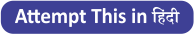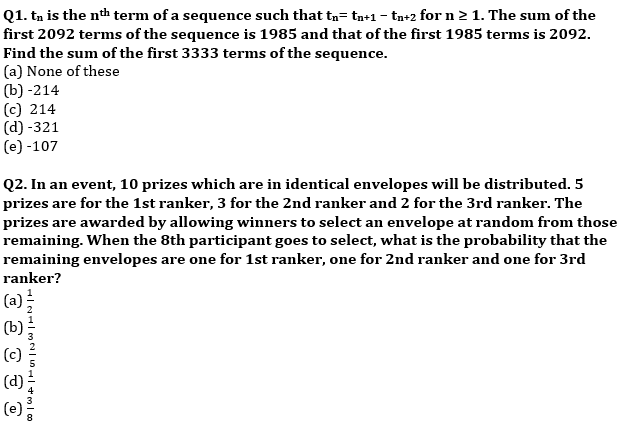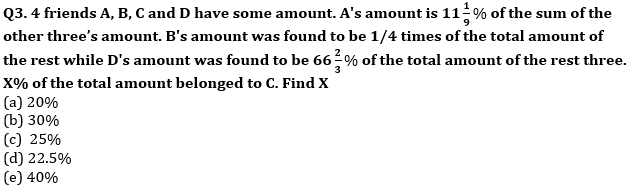Q4. A 5-digit number abcde is made by using only 2,3,4,5,6 such that its three-digit abc is divisible by 4, next three-digit bcd is divisible by 5 and next cde is divisible by 3. How many such no. are possible, if each digit of the number is different?
(a)4
(b)none of these
(c)2
(d)3
(e)1

Q5. Y is a natural number of 3 digits and its left most digit is 9. When this leftmost digit is removed from Y, the number thus obtained is found to be 1/21 times of Y. What is the sum of the all the digits of Y.
(a)18
(b)17
(c)16
(d)15
(e)none of these

There are six persons viz. A,B,C,D,E&F. A can complete a piece of work in ‘x’ days. B takes double the time taken by A, C takes double the time taken by B, D takes double the time taken by C, E takes double the time taken by D and F takes double the time taken by E to complete the same task.

Q6. If they are paired in groups of three each and first pair takes four-fifths the time needed by the second pair to complete the work, then which of the following pairs of persons can be the first pair?
(I) B,C&F
(II) A,B&C
(III) A, E &F
(a) only (III)
(b) only (II) and (III)
(c) only (I) and (III)
(d) All of the above
(e) only (I) and (II)

Q7. If they are paired in a group of two persons, then which pair among the below given pairs will take less than 1.5x days to complete the above work?
(I) A & D
(II) B & F
(III) C & B
(IV) D & E
(V) A & E
(a) only (IV) & (V)
(b) only (I), (II) and (III)
(c) only (I), (III) and (V)
(d) All of the above
(e) only (II) and (III)

Q8. Boat covers (X) km in downstream in Ganga in 10 hours and same boat covers (X) km in upstream in Yamuna in 18 hours. If speed of current in Ganga is 40% less than that of in Yamuna, then what can be the possible value (s) of speed of boat in still water and speed of current in Ganga.
(I) (24 & 6)
(II) (40 & 8)
(III) (32 & 8)
(IV) (30 & 7.5)
(V) (20 & 4)
(a)only (I), (III) and (IV)
(b)only (III), (IV) and (V)
(c)only (V)
(d) All of the above.
(e)only (II) and (V)

A man invested Rs.X at R% p.a. at CI compounding annually. If difference between 1st year CI and 2nd year CI is Rs.2592 and difference between 2nd year CI and 3rd year CI is Rs.2903.04.

Q9. Find value of R%.
(a) 10%
(b) 18%
(c) 14%
(d) 12%
(e) 16%

Q10. Find value of X.
(a) Rs.100000
(b) Rs.140000
(c) Rs.220000
(d) Rs.180000
(e) Rs.60000

Solutions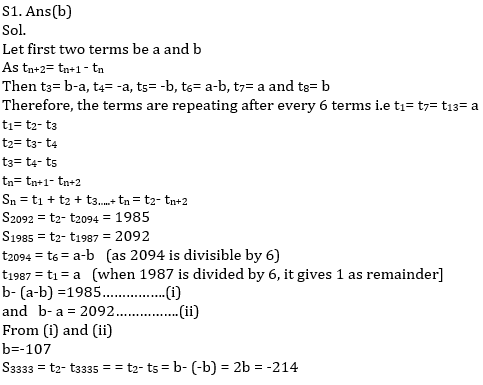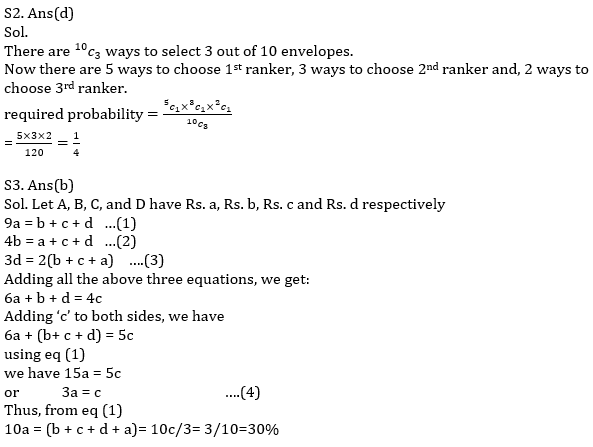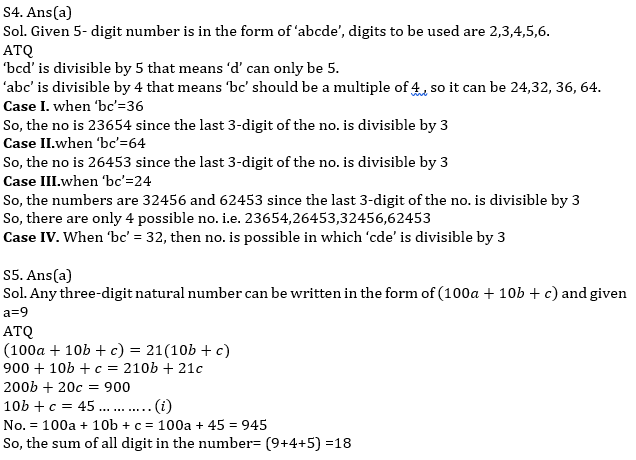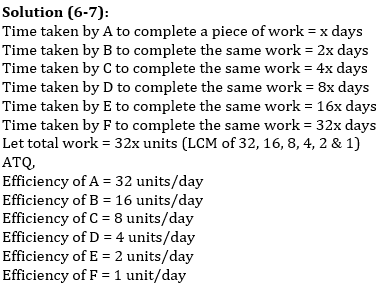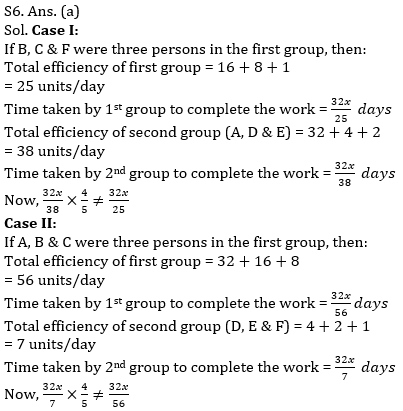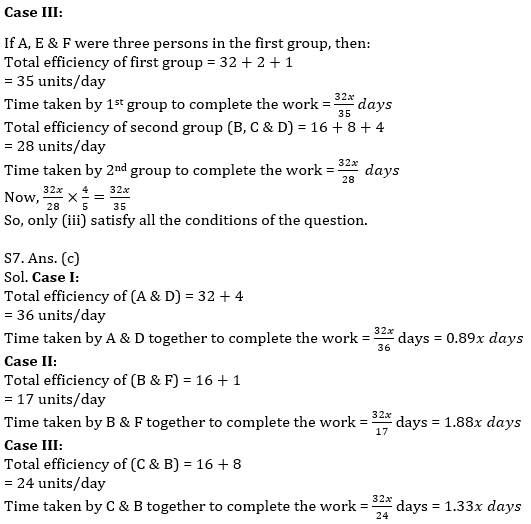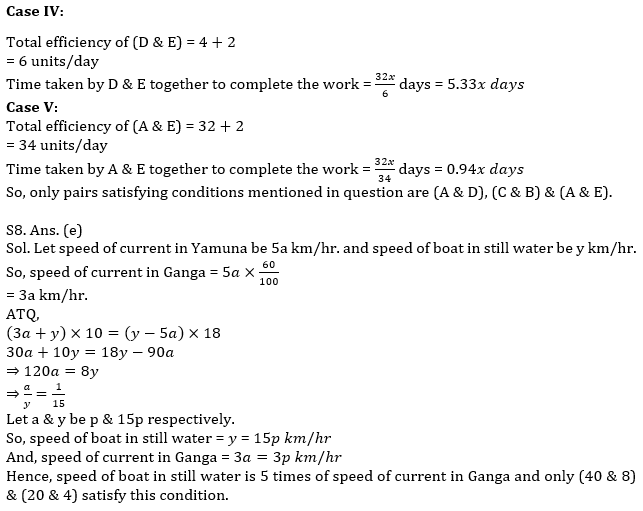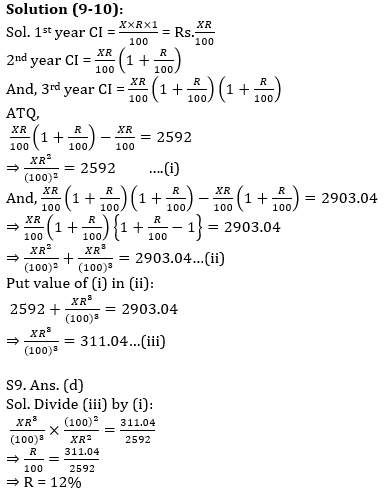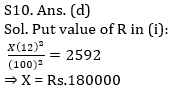#### Congratulations!Incorrect details? Fill the form again here

•Quantitative Aptitude Quiz For NABARD Gr...
•Quantitative Aptitude Quiz For NABARD Gr...
•Quantitative Aptitude Quiz For NABARD Gr...
•Quantitative Aptitude Quiz For NABARD Gr...
•Quantitative Aptitude Quiz For IBPS RRB ...
•Quantitative Aptitude Quiz For IBPS Cler...